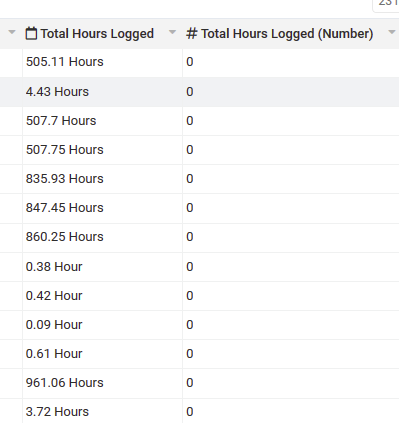# Sum Connected Date Total Hours Field

I’m completely lost, and apparently the first one to attempt this and fail. What I want to do is to sum the totals of a date formula field that calculates the # of hours worked.

So…

Object 1: Date Formula Field = x.xx hours

Object 2: Total Hours Logged = Sum of all connected records Date Formula Fields

I can find no way to accomplish this. I can’t get the date formula to populate a number field. I can’t sum the date formula field from the connected records, etc.

Anyone have any advice?

@Tophinity this is a great point.

Can you tell me how you’re getting the date formula values? Is it something like date2 - date1?

We’ll add this option natively.

But here’s perhaps an alternative to doing this. Requires using a pipe though.

1. Install the “Tadabase Date Utilities” pipe.
2. Inside your table rules (or record rules) add a rule with the pipe and use the “Subtract Times” method.
3. For the parameters, set the Datetime to the Start time and set the “End Time” to the end time. This will do the following: (Endtime - Datetime).
4. Next, in the Update Record set the Number field you have to the Pipe Value of “Total Hours”

This will make the value appear as a regular number:

You can then use that value as a sum in the parent table

Yes it is date/time 1 - date/time 2 = x.xx hours

Got it, thanks! I’ll try that. It would be awesome if I could just do that with normal field rules. The issues I’ve encountered have mostly been related to the inability to access date information with rules/formulas to pull the data I need.

Whether it’s pulling a date in with a text formula to display just the value, populating a number field such as here, or using standard date/time fields in conjunction with the date range fields.

I’ll try to use pipes to solve these types of problems going forward. Thanks @moe

Unfortunately, the pipes method doesn’t appear to be working at all @moe. As you can see, I’m trying to calculate it the same way, but I’m just getting values of 0.Another option would be to use a regular equation field, however it won’t allow me to use decimal places, leaving me with just whole numbers. I have to be missing something here.

Hi @Tophinity, hopefully this will help you. I have a similar time clock app that I made and the way I get around this is by adding a number field with the amount of decimals you would like and then on create and edit of every record I set the Static Number field to the equation decimal result.

I hope it helps.

I also round the decimal time to the nearest 15 minutes like this:
ROUND((({Minutes}/60)+{Hours})*4,0)/4

The work around allows me to use cards to display total hours and I’m sure whatever you are trying to do should work.The only problem with this solution is the equation has to run before the set rule which will only happen if the record is updated after the equation runs. The way I get around this is by running a task every morning that edits all the new records for the week. If you want more of a realtime update you will have to utilize integromat to do that. or if you can set the equation in a pipe that would also work.

1 Like

This is amazing @SuiteUpstairs. Thank you so much. I’m going to cycle back to this soon, and your help here is exactly what I need. You rock!1 Like